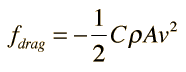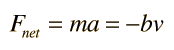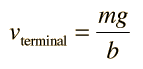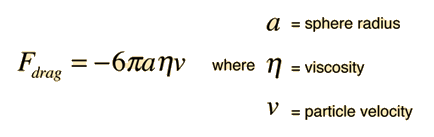الموضوع: Air Friction

1.Air Friction

Air friction, or air drag, is an example of fluid friction. Unlike the standard model of surface friction, such Friction forces are velocity dependent. The velocity dependence may be very complicated, and only special cases can be treated analytically. At very low speeds for small particles, air resistance is approximately proportional to velocity and can be expressed in the formwhere the negative sign implies that it is always directly opposite the velocity. For higher velocites and larger objects the frictional drag is approximately proportional to the square of the velocity:where ρ is the air density, A the cross-sectional area, and C is a numerical drag coefficient.
Linear Velocity Dependence

For objects moving at relatively low speeds through a liquid, where turbulence is not a significant factor, then the viscous resistance to the object's motion is approximately proportional to its velocity. Even in gases there are circumstances where the frictional resistance is approximately proportional to the velocity - such as the motion of tiny dist particles through the air. More commonly, air friction has terms proportional to the square or even higher powers of the velocity.
For linear velocity dependence the resistance force can be written
fresistance = -bv . If this resistance is the only force acting, then
Newton's second law becomesAnalyze motion
which will bring the object to rest in a distance.
If the weight W=mg of the object also acts, then the object approaches a terminal velocity :This terminal velocity is expressed in terms of an effective value of g if the buoyant force is significant.2.رد: Air Friction

Viscous Resistance

If an object moves slowly through a liquid, it will experience a viscous drag which is approximately proportional to the velocity. For a sphere falling through a liquid in a velocity regime where viscosity is the dominant effect and turbulence can be neglected, the viscous drag isThis resistance can be used to calculate penetration distance into a viscous medium, terminal velocity, and motion description for sinking objects.

Fluid Friction

The resistance to an object's motion through a fluid may be termed "fluid friction." It may take the form of viscous resistance in a liquid, or the rather different character of air friction when an object moves through a gas3.رد: Air Friction

بوركتم4.رد: Air Friction

شكرا لكم5.رد: Air Friction

شكرااااااااااا6.رد: Air Friction

أشكركم على هذا الانتقاء7.رد: Air Friction

جزاكم الله خير الجزاءصفحة 1 من 2 1 2 الأخيرةمعلومات الموضوع

الأعضاء الذين يشاهدون هذا الموضوع

الذين يشاهدون الموضوع الآن: 1 (0 من الأعضاء و 1 زائر)ضوابط المشاركة

• لا تستطيع إضافة مواضيع جديدة
• لا تستطيع الرد على المواضيع
• لا تستطيع إرفاق ملفات
• لا تستطيع تعديل مشاركاتك
•